### IMO Shortlist 1982 problem 18

Kvaliteta:
Avg: 0.0
Težina:
Avg: 0.0
Let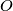$O$ be a point of three-dimensional space and let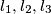$l_1, l_2, l_3$ be mutually perpendicular straight lines passing through$O$. Let$S$ denote the sphere with center$O$ and radius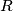$R$, and for every point$M$ of$S$, let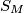$S_M$ denote the sphere with center$M$ and radius$R$. We denote by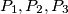$P_1, P_2, P_3$ the intersection of$S_M$ with the straight lines$l_1, l_2, l_3$, respectively, where we put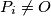$P_i \neq O$ if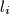$l_i$ meets$S_M$ at two distinct points and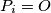$P_i = O$ otherwise (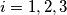$i = 1, 2, 3$). What is the set of centers of gravity of the (possibly degenerate) triangles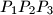$P_1P_2P_3$ as$M$ runs through the points of$S$?
Source: Međunarodna matematička olimpijada, shortlist 1982